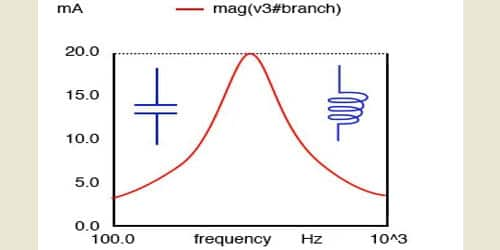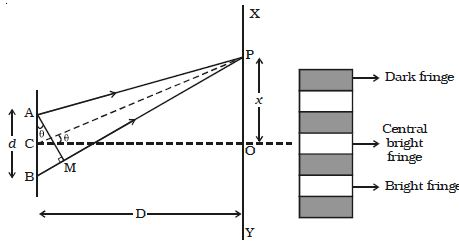# Describe Expression for Bandwidth with Equation

An important property of a resonant circuit is its bandwidth. Bandwidth is defined as the size of the frequency range that is passed or rejected by the tuned circuit. Another name for the tuning circuit is a resonant circuit. A resonant circuit has a specific frequency and bandwidth and we put that to use in the radio receiver.

Expression for bandwidth

Let d be the distance between two coherent sources A and B of wavelength λ. A screen XY is placed parallel to AB at a distance D from the coherent sources. C is the midpoint of AB. O is a point on the screen equidistant from A and B. P is a point at a distance x from O, as shown in Figure. Waves from A and B meet at P in phase or out of phase depending upon the path difference between two waves.Fig: Interference band width

Draw AM perpendicular to BP

The path difference δ = BP – AP

AP = MP

∴ δ = BP – AP = BP – MP = BM

In right angled Δ ABM, BM = d sin θ

If θ is small, sin θ = θ

∴ The path difference δ = θ.d

In right angled triangle COP, tan θ = OP/ CO = x/D

For small values of θ, tan θ = θ

The path difference δ = xd/D

Resonant can be obtained in either series or parallel circuits containing three electrical characteristics namely; resistance, inductance, and capacitance. The resonant circuit below consists of a resistor, an inductor and a capacitor in series with a current meter and a voltage source. The voltage source represented by an AC symbol is also variable in frequency. Most commonly, we use one particular AC voltage: 120 volts 60 Hz, but in this case, we’re very interested in the frequency being variable so it would be some value other than 60 Hz.